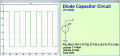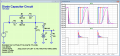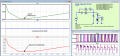# Help with diode and capacitor

#### felastian123

Joined Oct 10, 2021
9

#### Papabravo

Joined Feb 24, 2006
20,369
As your schematic stands you are using the "default" diode model. Depending on your understanding of this default model you may or may not get intuitive results when you run the simulation. It would be better for you to pick an actual diode model for at least two reasons:
1. Other people can run the simulation and help you understand what is happening
2. You will avoid having to understand the default diode
What does the "0a" next to the 10v on voltage source V1 supposed to do?
V1 does not have what looks like it has the correct specification for a square wave.
Is it possible there is no solution to this problem?

•felastian123

#### felastian123

Joined Oct 10, 2021
9
As your schematic stands you are using the "default" diode model. Depending on your understanding of this default model you may or may not get intuitive results when you run the simulation. It would be better for you to pick an actual diode model for at least two reasons:
1. Other people can run the simulation and help you understand what is happening
2. You will avoid having to understand the default diode
What does the "0a" next to the 10v on voltage source V1 supposed to do?
Is it possible there is no solution to this problem?
sorry, the diode that I use is the 1N4142, I understand that 0A 10V means the square signal begin in the 0v, and the peak is 10V, I can simplify the circuit understanding how the C1 works? Ty

#### Papabravo

Joined Feb 24, 2006
20,369
sorry, the diode that I use is the 1N4142, I understand that 0A 10V means the square signal begin in the 0v, and the peak is 10V, I can simplify the circuit understanding how the C1 works? Ty
I don't think you understand how to make a square wave source. This is what a proper square wave source should look like. I have used parameters so that the properties can be easily modified as you experiment with the simulation.

TT = Transition time = risetime + falltime = 40 nanoseconds
f = squarewave frequency
ontime + transition time = 0.5*(Period)
Other configurations are possible•felastian123

#### Papabravo

Joined Feb 24, 2006
20,369
I don't have a model of 1 1N4142, but using a 1N4148 small signal diode, and depending on your definition of "conducting", I do not believe this problem has a solution. For low values of C1's capacitance, the diode D3 conducts whenever D1 conducts. As the value of C1 increases you trade rise time and fall time for time at peak value. Reaching a steady state where D1 "conducts" 1/3 the time of D1 appears to be a challenge.•felastian123

#### felastian123

Joined Oct 10, 2021
9
I don't have a model of 1 1N4142, but using a 1N4148 small signal diode, and depending on your definition of "conducting", I do not believe this problem has a solution. For low values of C1's capacitance, the diode D3 conducts whenever D1 conducts. As the value of C1 increases you trade rise time and fall time for time at peak value. Reaching a steady state where D1 "conducts" 1/3 the time of D1 appears to be a challenge.

View attachment 277470
Thank you very much for the help!!!

#### Papabravo

Joined Feb 24, 2006
20,369
I have added a .measure script to the simulation to demonstrate that a 33% conduction time on D3 with respect to D1 is not achievable by a considerable margin. 64% is about as low as you can go. do you have any thoughts on what you might do to make the goal achievable? Changing the values of R1 and R2 perhaps.Direct Newton iteration for .op point succeeded.
.step ct=1e-006
.step ct=1e-005
.step ct=0.0001
.step ct=0.00047
.step ct=0.00068
.step ct=0.001
.step ct=0.0012
.step ct=0.0016
.step ct=0.002
.step ct=0.0022

Measurement: ts1r
step i(d3)=0.7ma
1 0.66711
2 0.670999
3 0.701833
4 1.06836
5 1.4059
6 1.74179
7 2.07552
8 2.40819
9 2.74015
10 3.0725

Measurement: ts1f
step i(d3)=0.7ma
1 0.833351
2 0.833505
3 0.835061
4 1.1748
5 1.51168
6 1.85062
7 2.18735
8 2.5274
9 2.86738
10 3.20392

Measurement: dt1
step ts1f-ts1r
1 0.166241
2 0.162506
3 0.133228
4 0.106434
5 0.105781
6 0.108835
7 0.111831
8 0.119209
9 0.127233
10 0.131428

Measurement: ts2r
step i(d1)=0.7ma
1 0.666667
2 0.666667
3 0.666667
4 0.666667
5 0.666667
6 0.666667
7 0.666667
8 0.666667
9 0.666667
10 0.666667

Measurement: ts2f
step i(d1)=0.7ma
1 0.833333
2 0.833333
3 0.833333
4 0.833333
5 0.833333
6 0.833333
7 0.833333
8 0.833333
9 0.833333
10 0.833333

Measurement: dt2
step ts2f-ts2r
1 0.166667
2 0.166667
3 0.166667
4 0.166667
5 0.166667
6 0.166667
7 0.166667
8 0.166667
9 0.166667
10 0.166667

Measurement: ratio
step dt1/dt2
1 0.997446
2 0.975039
3 0.799368
4 0.638602
5 0.634687
6 0.653011
7 0.670984
8 0.715252
9 0.763399
10 0.78857

#### felastian123

Joined Oct 10, 2021
9
I have added a .measure script to the simulation to demonstrate that a 33% conduction time on D3 with respect to D1 is not achievable by a considerable margin. 64% is about as low as you can go. do you have any thoughts on what you might do to make the goal achievable? Changing the values of R1 and R2 perhaps.
View attachment 277474
Direct Newton iteration for .op point succeeded.
.step ct=1e-006
.step ct=1e-005
.step ct=0.0001
.step ct=0.00047
.step ct=0.00068
.step ct=0.001
.step ct=0.0012
.step ct=0.0016
.step ct=0.002
.step ct=0.0022

Measurement: ts1r
step i(d3)=0.7ma
1 0.66711
2 0.670999
3 0.701833
4 1.06836
5 1.4059
6 1.74179
7 2.07552
8 2.40819
9 2.74015
10 3.0725

Measurement: ts1f
step i(d3)=0.7ma
1 0.833351
2 0.833505
3 0.835061
4 1.1748
5 1.51168
6 1.85062
7 2.18735
8 2.5274
9 2.86738
10 3.20392

Measurement: dt1
step ts1f-ts1r
1 0.166241
2 0.162506
3 0.133228
4 0.106434
5 0.105781
6 0.108835
7 0.111831
8 0.119209
9 0.127233
10 0.131428

Measurement: ts2r
step i(d1)=0.7ma
1 0.666667
2 0.666667
3 0.666667
4 0.666667
5 0.666667
6 0.666667
7 0.666667
8 0.666667
9 0.666667
10 0.666667

Measurement: ts2f
step i(d1)=0.7ma
1 0.833333
2 0.833333
3 0.833333
4 0.833333
5 0.833333
6 0.833333
7 0.833333
8 0.833333
9 0.833333
10 0.833333

Measurement: dt2
step ts2f-ts2r
1 0.166667
2 0.166667
3 0.166667
4 0.166667
5 0.166667
6 0.166667
7 0.166667
8 0.166667
9 0.166667
10 0.166667

Measurement: ratio
step dt1/dt2
1 0.997446
2 0.975039
3 0.799368
4 0.638602
5 0.634687
6 0.653011
7 0.670984
8 0.715252
9 0.763399
10 0.78857
Another data that I have is the time that take the capacitor to charge, that is the double of the period in V1 before the steady state, I think in maybe use the charge capacitor formule, I dont know if is correct get Vc final with V2 (4v) and the diode 3(I understand with the datasheet that 1n4142 have 1v voltage drop), and with this data obtain C1.

#### WBahn

Joined Mar 31, 2012
29,137
Hi community, I need to find the value of C1 for the time conduction of diode D3 be 1/3 the time conduction of the diode D1, I know that V1 is a square wave function, with 10v, 3 Hz and a work cycle of 50%, how I can face this problem? any kind of help is appreciated, ty and sorry for my english.View attachment 277467
What diode model are you expected to use when solving this problem? The ideal diode model? The constant-voltage diode model? The piecewise linear diode model? The exponential diode model?

What is the point of this exercise? By that I do not mean anything about finding the value of a capacitor. Instead, I mean what are you expected to learn or what skill are you expected to improve as a result of doing so? Two obvious possibilities come to mind. The first is to use a simulator and the second is to use analysis.

If the intent is to use a simulator, I hope it is more than just trying different values for C and hitting 'Run' each time, hoping that at some point you happen upon an acceptable answer. Instead, I hope that the intent is to set up a simulation that convincingly gets at the heart of the matter.

But, frankly, I think you would be much better served if the intent is to forget about simulators, at least initially, and analyze the circuit to answer the question.

The first thing is to sketch out what you expect the voltage on the capacitor to be over the course of one cycle of the 3 Hz waveform in steady state.

What are the asymptotic voltage levels on C1 -- in other words, if the frequency of V1 were slowed down enough, what are the minimum and maximum voltages you would see on C1?

Then go through and identify which diodes are conducting at different parts of that waveform. You may not be able to identify (yet) what time the diodes transition, but you should be able to identify the voltages at which they do.

Then you are in a position to greatly simplify the circuit for a detailed analysis during each of those regions.

Before you get started, however, look at that circuit and see if you can identify a component that can be removed because it has absolutely no effect on anything of interest in answering this question.

If you go through the process I've outlined above, you should discover some very interesting relationships that let you focus you attention on specific parts of the waveform.

•felastian123

#### WBahn

Joined Mar 31, 2012
29,137
Another data that I have is the time that take the capacitor to charge, that is the double of the period in V1 before the steady state, I think in maybe use the charge capacitor formule, I dont know if is correct get Vc final with V2 (4v) and the diode 3(I understand with the datasheet that 1n4142 have 1v voltage drop), and with this data obtain C1.
The 1n4142 has a maximum forward voltage of 1 V when it is conducting 3 A of current -- the typical forward voltage drop is quite a bit less (somewhere between 850 mV and 900 mV). Even if we take the 10 V of V1 and put it across the 680 Ω resistor directly we get less than 15 mA of current, which parks the typical forward voltage at between 650 mV and 700 mV, making the traditional 0.7 V forward diode drop a very reasonable estimate to use.

•felastian123

#### felastian123

Joined Oct 10, 2021
9
The 1n4142 has a maximum forward voltage of 1 V when it is conducting 3 A of current -- the typical forward voltage drop is quite a bit less (somewhere between 850 mV and 900 mV). Even if we take the 10 V of V1 and put it across the 680 Ω resistor directly we get less than 15 mA of current, which parks the typical forward voltage at between 650 mV and 700 mV, making the traditional 0.7 V forward diode drop a very reasonable estimate to use.
Thanks you very much !

#### WBahn

Joined Mar 31, 2012
29,137
Thanks you very much !
Please tell us how you are expected to work this problem? By analyzing the behavior manually? Or via simulation?

If you do it manually, you have some approximations that make it easy to get results. Specifically, you assume that the diode is not conducting if the voltage across it is anything less than Vd, whatever you choose that to be, and that it is conducting otherwise.

But in simulation, when is the diode conducting and when isn't it? In reality, a diode is going to conduct current if the forward voltage across it is anything greater than zero and the amount it conducts is a smooth increase, albeit exponential, making it difficult to define a specific point at which it starts conducting other than the point at which it is no longer reverse biased, but this leads to unreasonable results because the amount of conducted current is orders of magnitude below anything significant for much of that time. That makes it nearly impossible to clearly define what the conduction time is for D3.

But, you might be asking, shouldn't the manual calculations be close to the simulation results?

Yes, as long as the approximations aren't pushed too hard.

If the capacitor discharges to a voltage low enough that D3 is significantly reverse-biased, then the amount of time that it is operating in the "squishy" zone is limited and the results will be pretty close to the approximated expectations. But if the capacitor is only being discharged a small amount, perhaps not even enough to reverse bias D3, then it is conducting the entire time, albeit in subthreshold, and the actual results may be quite different than expected because the assumptions used weren't valid.

Last edited:
•felastian123

#### felastian123

Joined Oct 10, 2021
9
Please tell us how you are expected to work this problem? By analyzing the behavior manually? Or via simulation?

If you do it manually, you have some approximations that make it easy to get results. Specifically, you assume that the diode is not conducting if the voltage across it is anything less than Vd, whatever you choose that to be, and that it is conducting otherwise.

But in simulation, when is the diode conducting and when isn't it? In reality, a diode is going to conduct current if the forward voltage across it is anything greater than zero and the amount it conducts is a smooth increase, albeit exponential, making it difficult to define a specific point at which it starts conducting other than the point at which it is no longer reverse biased, but this leads to unreasonable results because the amount of conducted current is orders of magnitude below anything significant for much of that time. That makes it nearly impossible to clearly define what the conduction time is for D3.

But, you might be asking, shouldn't the manual calculations be close to the simulation results?

Yes, as long as the approximations aren't pushed too hard.

If the capacitor discharges to a voltage low enough that D3 is significantly reverse-biased, then the amount of time that it is operating in the "squishy" zone is limited and the results will be pretty close to the approximated expectations. But if the capacitor is only being discharged a small amount, perhaps not even enough to reverse bias D3, then it is conducting the entire time, albeit in subthreshold, and the actual results may be quite different than expected because the assumptions used weren't valid.
Im working this problem manually, I think in simplify the circuit assuming that when C1 is charging, the current dont flow in D3, and when C1 is discharging, the current flow for D2, I assume the Max voltage in C1 is 4.7, and I think in use the Charge capacitor equation, but I found a problem with the current, that value I dont have

#### WBahn

Joined Mar 31, 2012
29,137
Im working this problem manually, I think in simplify the circuit assuming that when C1 is charging, the current dont flow in D3, and when C1 is discharging, the current flow for D2, I assume the Max voltage in C1 is 4.7, and I think in use the Charge capacitor equation, but I found a problem with the current, that value I dont have
You are making a bad assumption about when current flows in the diodes. You are correct that the max voltage on C1 is 4.7 V, but that is because D3 is acting as a clamp, which requires that it be conducting. That means that once C1 reaches that point while charging via D1 that any further current through D1 is shunted by D3.

Show your work so that we can see where you are having your problem so that we can give you some hints.